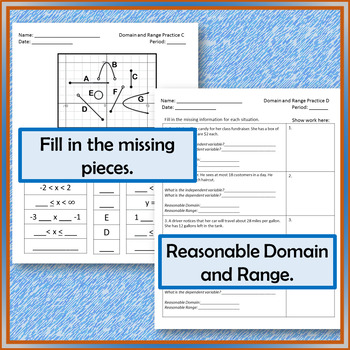# Domain and Range8th - 11th, Homeschool
Subjects
Standards
Resource Type
Formats Included
• PDF
•Activity
Pages
12 pages
Easel Activity Included
This resource includes a ready-to-use interactive activity students can complete on any device. Easel by TpT is free to use! Learn more.

### Description

Domain and range is a difficult concept for most algebra students. This resource is designed to practice this skill from graphs and word problems and to give the students some helpful tips on how to go about determining domain and range. This resources is a set of 4 worksheets and a Google Sheets presentation. The main focus of the presentation is to emphasis key elements of a graph to help determine domain and range.

• The first worksheet, Practice A, gives the students 10 graphs where the students are asked to find the domain and range. There are 5 linear graphs, 3 quadratic graphs, one circle and a graph with only points.
• Practice B is the opposite of the previous worksheet. It asks the students to create graphs with a given domain and range.
• Practice C gives the student a coordinate grid with multiple items graphed. The domain and range is listed, but with missing pieces that the students will complete.
• Practice D gives the students four situations where the they will need to determine the reasonable domain and range.
• All answer keys are provided.

Related Resource: Domain and Range Escape Boom Cards

Total Pages
12 pages
Included
Teaching Duration
2 days
Report this Resource to TpT
Reported resources will be reviewed by our team. Report this resource to let us know if this resource violates TpT’s content guidelines.

### Standards

to see state-specific standards (only available in the US).
Relate the domain of a function to its graph and, where applicable, to the quantitative relationship it describes. For example, if the function 𝘩(𝘯) gives the number of person-hours it takes to assemble 𝘯 engines in a factory, then the positive integers would be an appropriate domain for the function.
Understand that a function from one set (called the domain) to another set (called the range) assigns to each element of the domain exactly one element of the range. If 𝘧 is a function and 𝘹 is an element of its domain, then 𝘧(𝘹) denotes the output of 𝘧 corresponding to the input 𝘹. The graph of 𝘧 is the graph of the equation 𝘺 = 𝘧(𝘹).